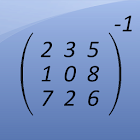Matrix Inversion Calculator

All Android applications categories

All Android games categories# Matrix Inversion Calculator

by: 507 7.8

7.8 Users
rating

## Screenshots

Description

This free app is a math calculator, which is able to calculate the invertible of a matrix.

The following matrices can be inverted:
- 2x2 matrices
- 3x3 matrices
- 4x4 matrices

Best math tool for school and college! If you are a student, it will helps you to learn linear algebra!
Note: The inverse of a matrix A is the matrix B, with AxB=I where I is the identity matrix and the multiplication used is ordinary matrix multiplication. A matrix is a nonsingular matrix if it is an invertible matrix, and the determinant of this matrix does not equal 0.

Tags: matrix inverse , find the inverse a 4x4 matrix , como invertir una matriz 2x2 java , how to invert a matrix in , matrix calculator apk , matriz widget , matrix invert , inverse of a matrix calculator 3x3 , inverted matrices

from 507 reviews

"Great"

7.8